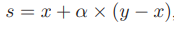# Balancing datasets using Crucio MWMOTE

Nowadays the majority of data sets in the industry are unbalanced. Meaning that a class has a higher frequency than others. Very often classifiers in such cases due to the unbalance of the data predict all samples as the most frequent class. To solve this problem we decided at Sigmoid to create a package that will have implemented all oversampling methods. We named it Crucio, and in this article, I will tell you about MWMOTE (Majority Weighted Minority Oversampling Technique).

## How does MWMOTE work?

Everything in MWMOTE starts with searching all minority samples with only k1-nearest neighbors as majority class samples. This helps to find the minority samples that are at the borderline between class space vectors. This set of samples is named: filtered minority set.

Next, for every sample in the filtered minority set, MWMOTE finds the k2-nearest neighbors of the majority class. Then it aggregates all these samples in a set of uniques samples. This set is named: borderline majority set.

Now for every sample from this set, we must find the k3-nearest neighbors from the minority class. This new set of uniques minority class samples is named: informative minority set.

Now using this Informative minority set we must compute the selection probability for every sample. But let’s begin with the reason for that.

Suppose we have the case illustrated below. Samples A and B are equally distant from the decision boundary. However the density of the majority class neighbors near A is higher than to B. This will make it more difficult for a classifier to learn A. Due to this, its selection probability will be higher.

To compute the selection probability we must first compute the selection weight by the following formulas.

Here CMAX and Cfth hyperparameters of MWMOTE.
Selection probability is just the normalized selection weight vector.

After computing the selection probabilities MWMOTE clusters the minority set with DBSCAN. The number of clusters is set as a hyperparameter.
Next MWMOTE selects using the selection probability a sample from a minority class, and another from the same cluster. Then the new sample is generated using the following formula.x and y are minority samples from the same cluster, alpha is a random number between 0 and 1.

## Using Crucio ICOTE.

In case you didn’t install Crucio yet, then type in the terminal the following:

``````pip install crucio
``````

Now we can import the algorithm and create the MWMOTE balancer.

``````from crucio import MWMOTE

mwmote = MWMOTE()
balanced_df = mwmote.balance(df, 'target')
``````

MWMOTE constructor has only 3 parameters:

• binary_columns (list, default=None) : if set to then it will check if the listed columns are in the passed data frame then they will be transformed to binary ones after generating new points.
• k1 (int, default = 5) : the number of neighbors used by KNN to find the filtered minority set.
• k2 (int, default = 5) : the number of neighbors used by KNN to find the borderline majority set.
• k3 (int, default = 5) : the number of neighbors used by KNN to find the Informative minority set.
• Cth (int, default = 5) : the threshold value of the closeness factor.
• CMAX (int, default = 2) : used in smoothing and rescaling the values of different scaling factors.
• M (int, default = 5) : the number of clusters found by DBSCAN.
• seed (int, default = 42) : seed for random number generation.

The balance function takes only 2 parameters:

• df (pd.DataFrame) : the pandas data frame that should be balanced.
• target (str) : the name of the column from the data frame that should be balanced.

Now let’s apply it to a data set. We will use it on the Pokemon dataset. The target column (Legendary) is unbalanced, only 8% is not legendary.
We recommend you, before applying any module from Crucio, to first split your data into a train and test data sets and balance only the train set. In such a way you will test the performance of the model only on natural data.
The results before balancing and after.

As you can see above, sometimes MWMOTE can get you some weird generated values. That’s why we highly recommend you play around with the parameters of the MWMOTE. For example in this case we set k1 = 3, k2 = 3, k3 = 3, M=2, Cth=3 and CMAX=3, so on small data sets, set the hyperparameters lower than the default ones.

However the accuracy is pretty good.

## Conclusion.

MWMOTE is a powerful method and, frankly speaking, a complex one. It needs a lot of finetunning, that’s why we highly recommend you to use it as a last solution, when other balancers don’t give the expected result.